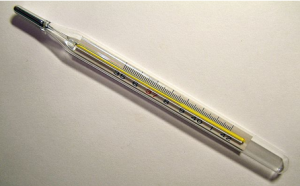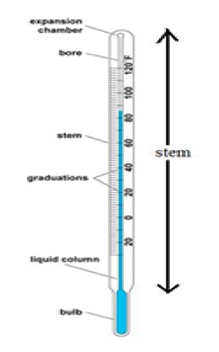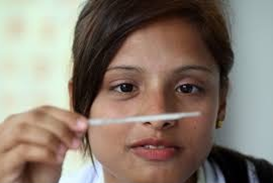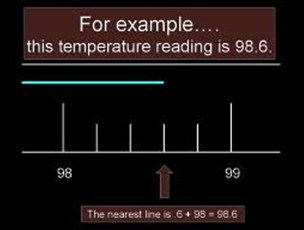In our day to day life, we come across objects that are hot or cold. Say, on a hot summer day we get relief from eating an ice cream and similarly in chilly winters from a cup of hot milk. Often, the need arises to find out how hot or how cold an object is, which we usually can find out by simply touching the objects. But we cannot always depend on our sense of touch to find out whether the object is hot or cold, as one may get hurt while touching a very hot object or the object may appear to be hot in one circumstance and relatively cold in the other. Water at normal temperature appears hot when compared to the ice while it feels cold when compared to boiling water. In order to find the exact measure of this hotness, the concept of temperature was introduced. Temperature is defined as the measure of the degree of hotness or coolness of an object. The device used for measuring temperature is called a thermometer.

### Thermometer:

We can recall, when somebody in the family suffered from fever, the temperature was measured by a thermometer. The thermometers that measure body temperature is called clinical thermometer.A thermometer looks like the one shown in the above figure. It consists of a long narrow uniform tube made of glass. It has a bulb at one end which contains mercury. Outside the bulb is a small shining thread of mercury. When we rotate the mercury we see two scales one with ⁰C and the other with ⁰F as the parameter.

#### Temperature Sensors in Thermometer:• The expansion of mercury due to heat is what tells us the temperature. If we calculate the expansion of mercury per degree change in temperature; then we can calibrate the thermometers with a scale based on the expansion. This is how a thermometer works. In honor of Fahrenheit’s discovery, we have named the temperature scale after him.
• The temperature sensors are so calibrated that the numbers on the thermometers represent the temperature of the body. Now we know that for any scale, we need the two reference points. The most obvious and sufficiently stable reference points are the boiling point and the melting point of water; being $100^{\circ}C$ and $0^{\circ}C$. At 100, the water boils over into the vapour state and at 0, the water melts from solid to liquid state.
• On the Fahrenheit scale, this would turn out to be $212^{\circ}F$ for the boiling point and $32^{\circ}F$ for the melting point. The Fahrenheit scale is used in the North America and a few other countries and the Celsius scale is used by the rest of the world. Below you will find the formulae for its conversion;
• $T_{c}=(T_{f}-32)\cdot \frac{5}{9}$

#### Steps:

• The thermometer is washed with water at normal temperature.
• It is given a few jerks. We notice that the jerks bring the level of mercury down. We make sure that it falls below the 37⁰C mark or the 98⁰F mark, the normal human body temperature.
• The thermometer has then kept under the tongue or under the arms in order to get the reading on the thermometer.
• The thermometer is then held close to the eye as shown in figure 3 in order to see the correct reading.• The temperature difference indicated between two bigger marks is noted. Also, the number of division (shown by smaller marks) between these marks is noted. For e.g. the bigger mark reads 10o and the number of division is 5, then each division reads the value equal to 10/5 = 2o• We note the value of the bigger mark that the mercury thread has crossed (which is 98 in the Fig.4 Example 1). Then the number of the division the mercury thread has crossed is noted (3 in this case). Now, this value is multiplied by the value of one division (which is 0.2 in this case). Hence, the temperature reading is 98 + (3×2) = 98.6.